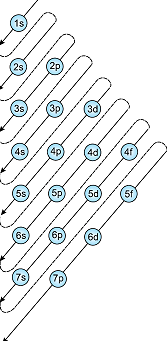# If n=6, the correct sequence for filling of electrons will be: Option 1) Option 2) Option 3) Option 4)

V Vakul

As we learnt in

Aufbau Principle -

In the ground state of the atoms, the orbitals are filled in order of their increasing energies.

- whereinIn the ground state of atoms, the orbitals are filled in order of their increasing energies.

Clearly for n=6, we have the order 6s, 4f, 5d, 6p or ns, (n-2)f, (n-1)d, np

Option 1)

This solution is correct

Option 2)

This solution is incorrect

Option 3)

This solution is incorrect

Option 4)

This solution is incorrect

Exams
Articles
Questions